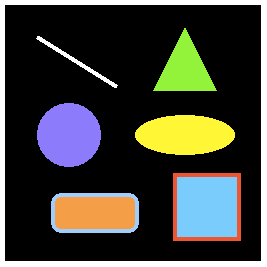## 用Pixi绘制几何图形### 矩形

let rectangle = new Graphics();

rectangle.beginFill(0x66CCFF);

rectangle.lineStyle(4, 0xFF3300, 1);

rectangle.drawRect(x, y, width, height);

rectangle.endFill();

let rectangle = new Graphics();rectangle.lineStyle(4, 0xFF3300, 1);rectangle.beginFill(0x66CCFF);rectangle.drawRect(0, 0, 64, 64);rectangle.endFill();rectangle.x = 170;rectangle.y = 170;app.stage.addChild(rectangle);

### 圆形

drawCircle(x, y, radius)

let circle = new Graphics();circle.beginFill(0x9966FF);circle.drawCircle(0, 0, 32);circle.endFill();circle.x = 64;circle.y = 130;app.stage.addChild(circle);

### 椭圆

drawEllipse是一个卓越的Canvas绘画api，Pixi也能够让你调用drawEllipse来绘制椭圆。

drawEllipse(x, y, width, height);

x/y坐标位置决定了椭圆的左上角（想象椭圆被一个不可见的矩形边界盒包围着-盒的左上角代表了椭圆x/y的锚点位置）。下面是50像素宽20像素高的黄色椭圆。

let ellipse = new Graphics();ellipse.beginFill(0xFFFF00);ellipse.drawEllipse(0, 0, 50, 20);ellipse.endFill();ellipse.x = 180;ellipse.y = 130;app.stage.addChild(ellipse);

### 圆角矩形

Pixi同样允许你调用drawRoundedRect方法来创建圆角矩形。这个方法的最后一个参数cornerRadius是单位为像素的数字，它代表矩形的圆角应该有多圆。

drawRoundedRect(x, y, width, height, cornerRadius)

let roundBox = new Graphics();roundBox.lineStyle(4, 0x99CCFF, 1);roundBox.beginFill(0xFF9933);roundBox.drawRoundedRect(0, 0, 84, 36, 10)roundBox.endFill();roundBox.x = 48;roundBox.y = 190;app.stage.addChild(roundBox);

### 线段

let line = new Graphics();line.lineStyle(4, 0xFFFFFF, 1);line.moveTo(0, 0);line.lineTo(80, 50);line.x = 32;line.y = 32;app.stage.addChild(line);

PIXI.Graphics对象，比如线段，都有xy值，就像精灵一样，所以你可以在绘制完它们之后将他们定位到舞台的任意位置。

### 多边形

let path = [  point1X, point1Y,  point2X, point2Y,  point3X, point3Y];graphicsObject.drawPolygon(path);

drawPolygon会将上面三个点连接起来创造图形。下面是如何使用drawPolygon来连接三条线从而创建一个红底蓝边的三角形。我们将三角形绘制在（0，0）的位置上，之后通过调整它的xy属性来移动它在舞台上的位置。

let triangle = new Graphics();triangle.beginFill(0x66FF33);//Use drawPolygon to define the triangle as//a path array of x/y positionstriangle.drawPolygon([    -32, 64,             //First point    32, 64,              //Second point    0, 0                 //Third point]);//Fill shape's colortriangle.endFill();//Position the triangle after you've drawn it.//The triangle's x/y position is anchored to its first point in the pathtriangle.x = 180;triangle.y = 22;app.stage.addChild(triangle);